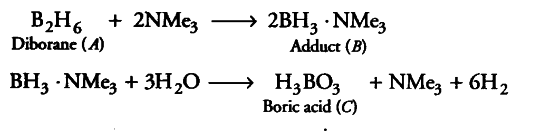# A compound (A) of boron reacts with \$NMe_{ 3}\$ to give an adduct

A compound (A) of boron reacts with \$NMe_{ 3}\$ to give an adduct (B) which on hydrolysis gives a compound © and hydrogen gas. Compound © is an acid. Identify the compounds A, B and C. Give the reactions involved.

Since, compound ‘A ’ of boron reacts with NMe_{ 3} to form an adduct ‘B’. Thus, compound ‘A’ is a Lewis acid. Since, adduct ‘ B’ on hydrolysis gives an acid ‘C’ and hydrogen gas, therefore, ‘ A’ is \$B_{ 2}\$\$H_{ 6}\$ and ‘C is boric acid.